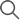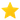# What Is The Probability Of Drawing A Spade Face Card?

Many believe that playing card games is all about luck and practice. Well, that might not be entirely true. It is quite accurate that you need both practice and luck, but those are not the only factors that contribute towards your victory. Most part of it is logic and mathematics, and the winning concoction is a proportionate mix of all the factors mentioned above. Believe it or not, math textbooks now have a ton of problems on playing cards and finding various probabilities, making learning easier for kids of this day and age. Not only do you have an upper hand if you are good with mathematics, but will also let you calculate your opponent’s gameplay, ultimately paving a victorious path for you (with the right amount of luck, of course). In this post, we will tell you about the probability of getting a spade face card. Before that, let’s brief you about the basics of a deck of cards.

A regular deck of 52 cards are divided into 4 suites, each consisting of 13 cards. The four suites are namely hearts, clubs, diamonds and spades. Each suite has ace, 2, 3, 4, 5, 6, 7, 8, 9, 10, jack, queen and king. These are the most common packs of playing cards today. Face cards are ideally the cards in a deck which has pictures or figures in them (that is, they have a face depicted in them). Hence the face cards are king, queen and jack, all of which have faces shown on them. The rules and different nooks of these playing cards are well known but very rarely do people question the whole system and numbering of the cards. Fun fact, the four suites in the deck are supposed to represent the four seasons we experience, the 52 cards signify the 52 weeks in a year and the thirteen cards present in a suite are said to be a representation of the thirteen lunar cycles we go through.

Making a callback to the mathematics part of playing cards, the probability of drawing any random card out of the deck is 1/52. The probability of drawing any one of the four suites calculated to be 13/52 which can be simplified as ¼, which is a much greater fraction than the initial probability of 1/52. Each suite has 3 face cards, meaning that there is a total of 12 face cards in the whole 52 cards in the deck. Now, drawing a face card out of the bunch would be a probability of 12/52.

The formula for the conditional probability of event A happening given that it's known event B already happened is given by the formula:

P(A∣B) = P(A∩B)/ P(B)

If we take A to be = "Drawing a face card" and B = "Drawing a spade", we can calculate the probability of this by finding two values: P(A∩B), or the probability of drawing a face card which happens to also be a spade card, and P(B), or the probability of drawing a spade out of the 52 cards.

Since there are three face cards in total in the deck (Jack, Queen, and King) or particularly in the spades suit, and 52 total possible cards, the P(A∩B) = 3/52. In a similar sense, we also know that there are 13 spades in a deck of 52 cards, so P(B) = 13/52 which can be simplified to be ¼.

Therefore:

P(A∣B) = P(A∩B)/ P(B) = (3/52) (13/52) = 3/13

Therefore, it can be positively concluded that the probability of drawing a spade face card is 3/13. In an alternative fashion, if one had to do this computation in a simple fashion, they can just take hold of the number of face cards in the whole of spade cards. Knowing that there are only 13 spade cards, out of which 3 are face cards, hence the probability can be calculated as 3/13.

Now that you know the probability of getting a spade face card, you can use this knowledge to determine the odds of flopping a spade face card in a real game and strategize accordingly. To practice poker and play poker online, download the GetMega app and register in any of the various contests.

## +4,409## What Our Players Feel

Get Android app link through SMSGetMega4.7 Rating